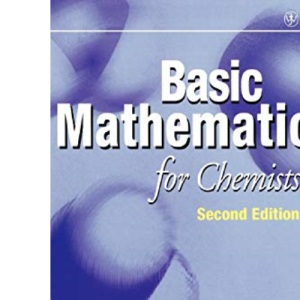30 Day Refund Guarantee# Basic Mathematics for Chemists 2e

by PeterTebbutt (Author)

##### Synopsis

Basic Mathematics for Chemists aims to teach the maths that chemists need to know through the use of applications, data, examples and problems all drawn from chemistry. The author demystifies the maths, and shows how, where and why it is used in chemistry. The text assumes little prior knowledge of maths and starts from basic mathematical principles, including understanding equations, notation, basic functions and their priorities. It then covers more specialised functions such as logarithms and trigonometric functions before presenting chapters on calculus. In this edition, there is a new chapter on vectors and matrices. FEATURES Written by a chemist for chemists .Many examples, problems and applications. Gentle introduction to the maths chemists needs to know New chapter on vectors and matrices. Fully worked examples and problems provided within each chapter CONTENTS: Preface; Equations, Functions and Graphs; Special Functions; Practical Statistics; Differential Calculus; Integral Calculus; Differential Equations; Statistics for Theoretical Chemistry; Complex Numbers, Vectors, Determinants and Matrices; Appendix 1: The Greek Alphabet; Appendix 2: Numerical Answers to Problems.

### \$72.80

Quantity

1 in stock

##### More Information

Format: Paperback
Pages: 292
Edition: 2nd Edition
Publisher: Wiley-Blackwell
Published: 27 Mar 1998

ISBN 10: 0471972843
ISBN 13: 9780471972846

##### Author Bio

Peter Tebbutt is the author of Basic Mathematics for Chemists, 2nd Edition, published by Wiley.